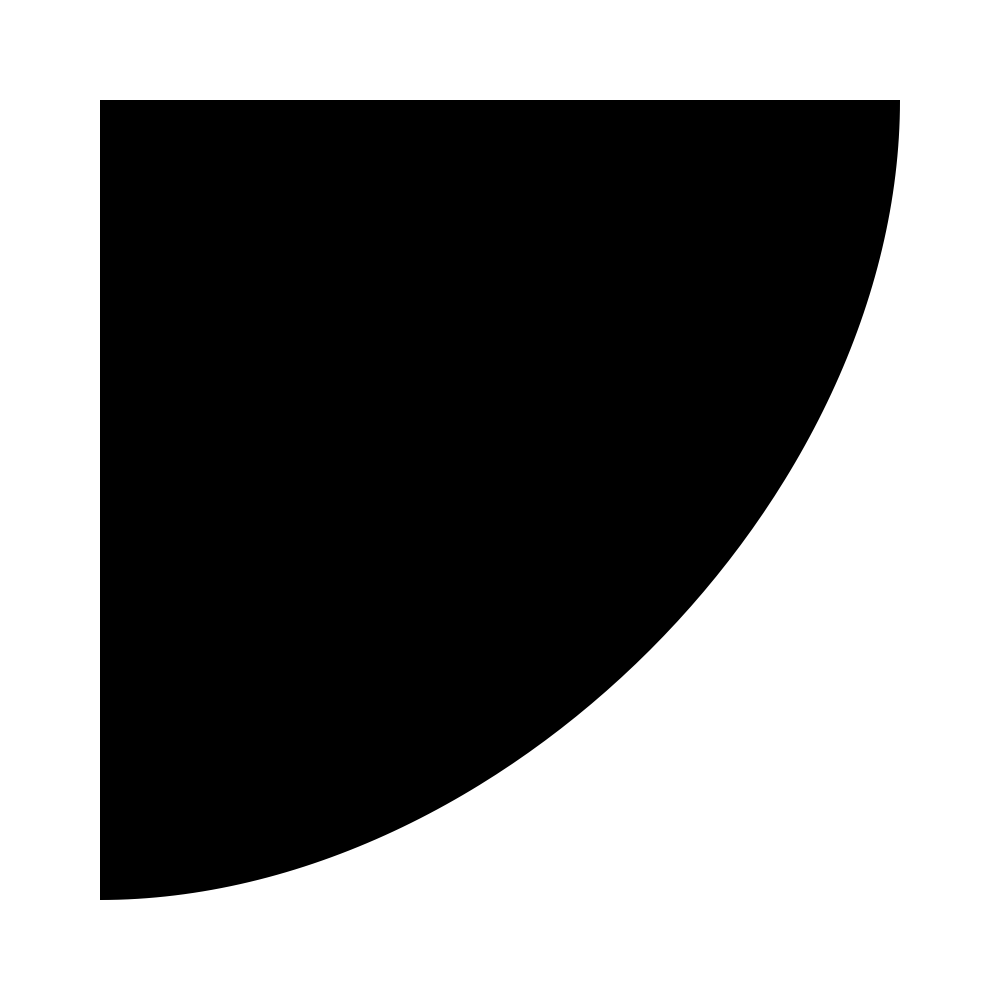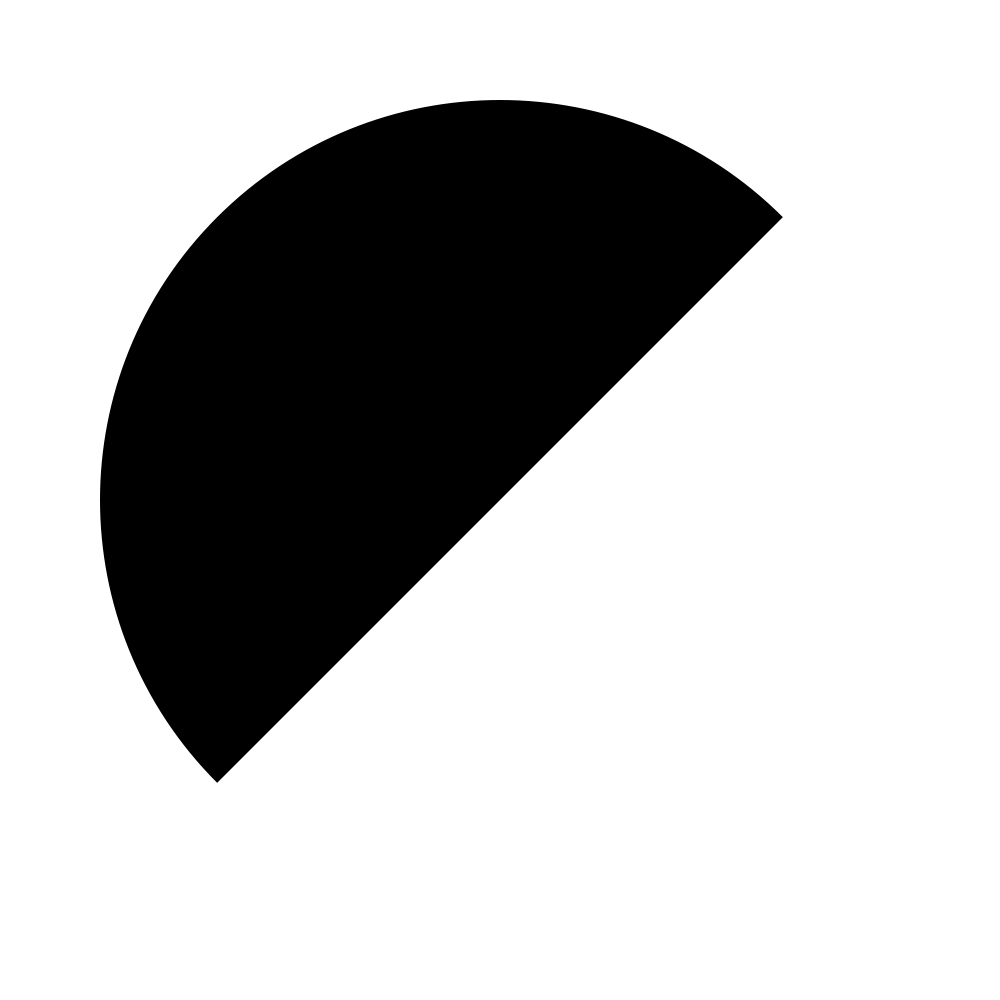# Drawing Paths¶

Using bezier paths.

`newPath`()

Create a new path.

`moveTo`(xy)

Move to a point x, y.

`lineTo`(xy)

Line to a point x, y.

`curveTo`(xy1, xy2, xy3)

Curve to a point x3, y3. With given bezier handles x1, y1 and x2, y2.

`qCurveTo`(*points)

Quadratic curve with a given set of off curves to a on curve.

`arc`(center, radius, startAngle, endAngle, clockwise)

Arc with center and a given radius, from startAngle to endAngle, going clockwise if clockwise is True and counter clockwise if clockwise is False.

`arcTo`(xy1, xy2, radius)

Arc from one point to an other point with a given radius.

```pt0 = 74, 48
pt1 = 238, 182
pt2 = 46, 252

def drawPt(pos, r=5):
x, y = pos
oval(x-r, y-r, r*2, r*2)

size(300, 300)
fill(None)

path = BezierPath()
path.moveTo(pt0)

stroke(0, 1, 1)
polygon(pt0, pt1, pt2)
for pt in [pt0, pt1, pt2]:
drawPt(pt)

stroke(0, 0, 1)
drawPath(path)
stroke(1, 0, 1)
for pt in path.onCurvePoints:
drawPt(pt, r=3)
for pt in path.offCurvePoints:
drawPt(pt, r=2)
```
`closePath`()

Close the path.

`drawPath`(path=None)

Draw the current path, or draw the provided path.

```# create a new empty path
newPath()
# set the first oncurve point
moveTo((100, 100))
# line to from the previous point to a new point
lineTo((100, 900))
lineTo((900, 900))

# curve to a point with two given handles
curveTo((900, 500), (500, 100), (100, 100))

# close the path
closePath()
# draw the path
drawPath()
````clipPath`(path=None)

Use the given path as a clipping path, or the current path if no path was given.

Everything drawn after a clipPath() call will be clipped by the clipping path. To “undo” the clipping later, make sure you do the clipping inside a with savedState(): block, as shown in the example.

```# create a bezier path
path = BezierPath()
# draw a triangle
# move to a point
path.moveTo((100, 100))
# line to a point
path.lineTo((100, 900))
path.lineTo((900, 900))
# close the path
path.closePath()
# save the graphics state so the clipping happens only
# temporarily
with savedState():
# set the path as a clipping path
clipPath(path)
# the oval will be clipped inside the path
oval(100, 100, 800, 800)
# no more clipping here
```